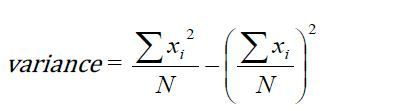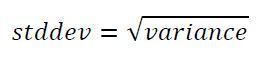Mean, Variance and Standard Deviation in PythonPosted by Samath9958 March 04, 2015

Write a function mean that takes a list and returns its mean value which is the sum of the values in the list divided by the length of the list. Function mean should use higher order procedure sumlist to calculate the sum and the function len to calculate the length of the list. If the list is empty return 0.

>>>mean([30,20,50,20])
30

```def sumfunc(num):
return num

def sumlist(mylist, sumfunc):
sum = 0
for i in mylist:
sum = sum + sumfunc(i)
return sum

def mean(mylist):
meanval = 0

if(len(mylist) == 0):
return 0
else:
meanval = sumlist(mylist,sumfunc)/len(mylist)
return meanval```

Write a function std_dev that takes a list of numbers and returns its standard deviation.
Variance is calculated by using the following formula:and standard deviation is square root of varianceFor example,
variance([10,20,30,40]) = ([102+202+302+402]/4) –(mean([10,20,30,40]))2
std_dev([10,20,30,40]) = math.sqrt(125)

Code:

```def variance(mylist):

mymean = mean(mylist)
mylen = len(mylist)
temp = 0

for i in range(mylen):
temp += (mylist[i] - mymean) * (mylist[i] - mymean)
return temp / mylen;

def std_dev(mylist):
return math.sqrt(variance(mylist))```